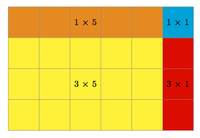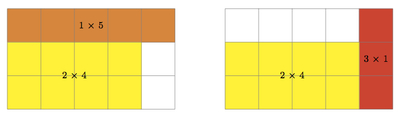# Distributive Property of Multiplication

Alignments to Content Standards: 7.NS.A.2.a

Lucia uses the picture below to explain the distributive property for the expression (3+1) $\times$ (5+1):1. Find (3+1) $\times$ (5+1) using the distributive property.
2. Explain how Lucia's picture relates to your calculation in (a).
3. How can Lucia use a picture to find (3-1) $\times$ (5-1) using the distributive property? Explain.

## IM Commentary

The goal of this task is to study the distributive property for products of whole numbers, focusing on using geometry to help understand why (-1) $\times$ (-1) = 1. This important property can be viewed as an application of the distributive property to whole numbers. While it is not easy to ''visualize'' taking a product of negative numbers, the pictures studied here add some tangible evidence why (-1) $\times$ (-1) = 1. A more abstract version of this task, dealing with products of more general negative numbers, can be found here: https://www.illustrativemathematics.org/tasks/1667.

Understanding of the distributive property begins in 3.OA.5 and 5.OA.5 as a means to help calculate products of whole numbers: in these settings, only addition is allowed. It continues to be important in high school and pictures like the ones used here provide valuable insight into the quadratic formula: see https://www.illustrativemathematics.org/illustrations/1827.

## Solution

1. Using the distributive property several times we find \begin{align}(3+1) \times (5 +1) &=  3 \times (5+1) + 1 \times (5+1) \\ &= (3 \times 5 + 3 \times 1) + (1 \times 5 + 1 \times 1) \\ &= (15+3) + (5+1)\\ &= 24.\end{align}
2. Below, each of the four rectangles making up the larger 4 by 6 rectangle is labeled:The four labeled rectangles do not overlap so the sum of their areas is the sum of the large rectangle. This tells us that $4 \times 6 = 3 \times 5 + 3 \times 1 + 1 \times 5 + 1 \times 1$ as we also saw in part (a).

3. One way to use the picture to evaluate $2\times 4$ would be to reverse the process of the previous part.  That is, a $2\times 4$ rectangle is a $3\times 5$ rectangle, minus a $1\times 4$ horizontal rectangle, minus a $2\times 1$ vertical rectangle, and finally, minus the $1\times 1$ square in the top right.  But the expression we get from this:  $$(3-1)\times (5-1)=3\times 5-4\times 1-2\times 1-1$$is not the distributive property.  So we look for another way of thinking about this.

First we draw a picture, color coded in a similar way, showing two copies of 3 by 5 rectangle containing a smaller (3-1) by (5-1) rectangle:Here the yellow rectangle is (3-1) by (5-1) while the larger surrounding rectangle is 3 by 5. We can get the 2 by 4 rectangle by taking away the orange and red rectangles from the 3 by 5 rectangle. But then we have taken away the top right square twice so we need to add it back in once. Putting in the areas of these rectangles we find $$(3-1) \times (5-1) = 3 \times 5 -3 \times 1 - 1 \times 5 + 1.$$ Expanding the left hand side of this equation using the distributive property as in (a) gives us $$(3-1) \times (5-1) = 3 \times 5 + 3 \times (-1) + (-1 \times 5) + (-1 \times -1).$$ Since $3 \times (-1) = -3 \times 1 = -3$ we deduce that the last terms in these two expressions are equal, that is $-1 \times -1 = 1$.